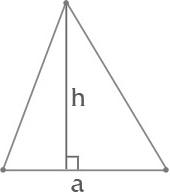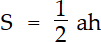Remove from favorites

# Area of a triangle, equilateral isosceles triangle area formula calculator

Area of a triangle, equilateral isosceles triangle area formula calculator allows you to find an area of different types of triangles, such as equilateral, isosceles, right or scalene triangle, by different calculation formulas, like geron's formula, length of triangle sides and angles, incircle or circumcircle radius.

## Method of calculating the area of a triangle

Base:    Height:Triangle is a polygon with three vertices, not lying on a one line, connected with three edges.
Formula for area of a triangle:,
where a - sides of a triangle, α - height